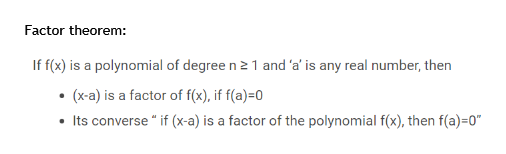Question
2 views

Find m so that x+5 is a factor of 5x3+29x2+mx+20

check_circle

Step 1

Let f(x)=5x^3 + 29x^2 +mx+20 , if (x+5) is a fact...help_outlineImage TranscriptioncloseFactor theorem: If f(x) is a polynomial of degree n 2 1 and 'a' is any real number, then (x-a) is a factor of f(x), if f(a)=0 Its converse " if (x-a) is a factor of the polynomial f(x), then f(a)=0" fullscreen

### Want to see the full answer?

See Solution

#### Want to see this answer and more?

Solutions are written by subject experts who are available 24/7. Questions are typically answered within 1 hour.*

See Solution
*Response times may vary by subject and question.
Tagged in

### Algebra